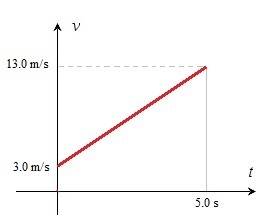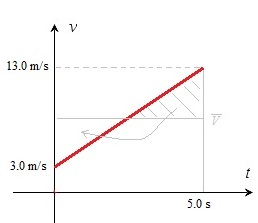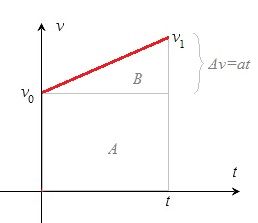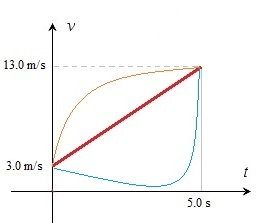# Formulas for the displacement, starting at non zero velocity

Up a level : Pre DP, Mechanics and Electronics
Previous page : Formulas for the displacement, starting at zero velocity
Next page : Free fallStarting at non zero velocity

Let us start with an example. We have an object that initially moves at 3.0 m/s, and after 5.0 s moves at 13.0 m/s. How far has it moved.

First we draw a v/t-diagam over the situation. It does not need to be exact or to scale.To find the area under the curve we split it into a rectangle and a triangle.We thus get 3·5=15 m for the rectangle and (13-3)·5/2=10·5/2=25 m for the triangle. That would give us 15+25=40 m in total.

Another way to do this is to look at the average velocity.  To use that would be as to cut of the top part of the triangle an fill it in to make a rectngle as in the figure below.We could thus use (13+3)/2=16/2=8 m/s, and the average velocity times the time=8·5=40 m.

Formulas

Let us again start at some non zero velocity, let us call it v0 and end at some higher velocity v1 as in the diagram.As before we can find the displacement by splitting the area under the curve into  two parts, a rectangle with the area A and a triangle with the area .The area A is easy to find: A=v0t.   For the area B we need to find the height of the triangle. Since we have that$\bar v = \frac{{\Delta s}}{{\Delta t}}$$a = \frac{{\Delta v}}{{\Delta t}}$

we get that$\Delta v = a\Delta t$

I.e. the change of velocity is the acceleration times the change of time. That sould make sense since acceleration is the rate of change of velocity, that is, a number of m/s every second, and then we multiply that by the number of seconds that has passed.   In this case the Δt is the time t, so we get$\Delta v = at$

So we get that$B = \frac{{{\text{base}} \cdot {\text{height}}}}{2} = \frac{{t \cdot at}}{2} = \frac{{a{t^2}}}{2}$

I.e. the same formula as for the displacement for the acceleration from 0. We add these to get the total displacement$s = {v_0}t + \frac{{a{t^2}}}{2}$

Let us try that with the example above. We have v0=3.0 m/s, To use the formula we need to find the acceleration. We get$a = \frac{{\Delta v}}{{\Delta t}} = \frac{{13 - 3}}{5} = \frac{{10}}{5} = 2\;{\text{m/}}{{\text{s}}^2}$

From this we now get$s = {v_0}t + \frac{{a{t^2}}}{2} = 3 \cdot 5 + \frac{{2 \cdot {5^2}}}{2} = 15 + 25 = 40\;{\text{m/s}}$

Let us instead look at the average velocity. We have that$\bar v = \frac{{\Delta s}}{{\Delta t}}$

From this we get that$\Delta s = \bar v\Delta t$

that, if we start at t=0 and at the origin (s0=0 m), becomes$s = \bar v t$

From this we get that$s = \frac{{{v_1} + {v_0}}}{2} \cdot t$

I would not remember this as a formula since it is such a direct consequence of that the displacement is the average velocity times the time.

A little bit of warning: You cannot use the above formula if you just happen to know thr initial velocity and the end velocity and the time taken. It will only work if you have a constant acceleration.  In the graph below you have three cases where the starting velocity is 3 m/s and the end velocity is 13 m/s. But the area under the curves=the displacements is definitely not the same.My suggestion is that you basically always start with drawing a v/t-diagram over the situation in problems dealing with velocity -acceleration -displacement. It will greatly decreases the risk of errors in your calculation. The v/t-diagram need not be exact, just a rough sketch  that would give you an overview of the problem often suffice.Up a level : Pre DP, Mechanics and Electronics
Previous page : Formulas for the displacement, starting at zero velocity
Next page : Free fallLast modified: Jan 31, 2017 @ 21:31